Actualisation

### Captures d’écran iPhone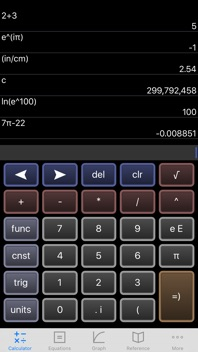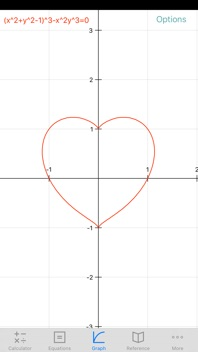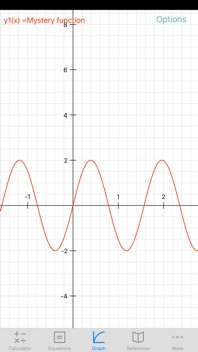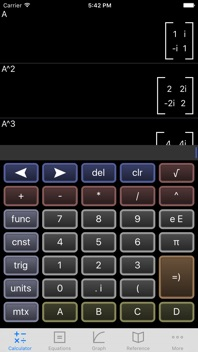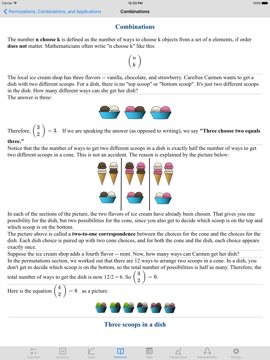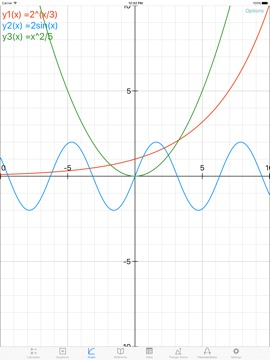### Description

The EduCalc Classic app, rewritten from the ground up:
-- All screens have portrait and landscape
-- Polynomial solver up to 10th degree
-- Wide range of settings for font sizes and graph line widths
-- Updates to this version will be more frequent, going forward
-- Matrix calculator more intuitive and is integrated with main calculator screen
-- Table has an "ask" mode where you enter input values and it gives you the outputs.
Features:
1) Scientific Calculator. A lot of thought has gone into the design of the keyboard, which gives you everything you need while keeping the keys as large as possible. Available functions include the following:
• the usual arithmetic functions and exponentiation.
• square root, cube root, nth root, natural log, log base 10, log of arbitrary base, absolute value, factorial, permutations (nPr), combinations (nCr), modulus, random integer, bell curve, cumulative normal distribution.
• Define and name your own constants
• Summations
• Complex numbers!
• Sig figs (enable under settings)
• Programmable keys (enable under settings)
2) Graphing. Capabilities:
• Graph up to nine equations at once.
• Graphs are labeled.
• You can drag the graph or pinch to zoom in or out.
• Calculator can find roots and intersections.
• Can graph in polar coordinates.
• Can graph parametric equations.
• Can graph parametric equations.
• Can graph implicit functions, such as x^2+y^2-4=0. Most calculator apps can't do this!
3) Vector and Matrix calculator. Vector dot and cross products. Multiply and add matrices. Determinant, transpose, Eigenvalues and Eigenvectors.
4) It can make a table of the values of any function you care to enter. You can choose the starting x value of the table, as well as how much x increases for each successive row.
5) Triangle solver. Enter three sides, two sides and an angle, or two angles and a side, and the solver calculates the rest.
6) Polynomial solvers. Find the roots of a polynomial, up to 10th degree.
7) System of linear equations solver.
8) The reference hits the high points of the following subjects:
algebra, calculus, geometry, trigonometry, vectors, vector calculus, and classical mechanics. So if you need a reminder of a trig identity . . . or the quadratic formula . . . or the chain rule from calculus . . . and so on, it's right there for you.
9) A unit converter. Your data are in meters, and you need an answer in feet? No problem! With a tap, you can enter the result of your conversion into the calculator. Currently converts different units of the following: acceleration, angle, area, density, distance, energy, force, mass, power, pressure, speed, temperature, time, and volume. Great for physics problem sets! You can also define your own units.
10) Constants for scientific calculations -- speed of light, strength of gravity at Earth's surface, etc. etc. etc. Tapping on a constant will insert it into your calculation -- i.e, you don't have to key in the value. Again, great for physics problem sets!
11) Statistics mode, including options for scatter plots and for regression lines.
12) Works on both iPhone and iPad.
13) Derivatives! (Answer simplification is limited.)
I'd love to learn about your comments or suggestions. You can write me at the email address on the settings screen of the app. Thanks!

### Nouveautés de la version 10.5

bug fix in Days between Dates
Fixed bug where function displayed on graph screen sometimes failed to update
updated lots of constants for 2019 redefinition (https://en.wikipedia.org/wiki/2019_redefinition_of_the_SI_base_units)

William Jockusch

### Informations

10.5

100,3 MB

Non

Oui

#### Classement

Classification : 4+

#### Compatibilité

iOS 9.0 ou plus récent

anglais

1 note

Pas d'entrée